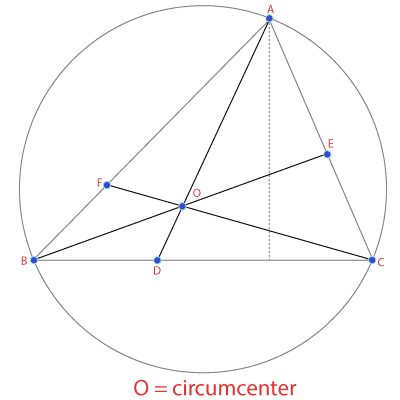# Circumcenter Calculator

Enter Information

#### Results

Fill the calculator form and click on Calculate button to get result here

Get Circumcenter Calculator For Your Website

Circumcenter calculator is used to calculate the circumcenter of a triangle by taking coordinate values for each line. It makes the process convenient by providing results on one click. You may find the manual calculation of circumcenter very difficult because it involves complicated equations and concepts. Circumcenter calculator takes values from you as input and gives you accurate results in a few seconds.

In this post, we will define circumcenter, discuss how to find circumcenter, and how to use our calculator to find circumcenter.

## How to use the circumcenter calculator?

Are you trying to calculate the circumcenter of a triangle for your class assignment? Follow these steps to find circumcenter using the above calculator.

• Enter the value of x and y for line
• Enter the value of x and y for line
• Enter the value of x and y for line
• Press the Calculate button to see the results.

It will instantly provide you with the values for x and y coordinates after creating and solving the equation. These values represent the circumcenter of a triangle, or in simple words, these values are the coordinates of the crossing point of perpendicular bisectors of a triangle.

Need to calculate the area of the triangle before calculating the circumcenter? Use our triangle area calculator.

## What is Circumcenter?

The circumcenter is the point at which the perpendicular bisectors of a triangle cross each other. It lies outside for an obtuse, at the center of the Hypotenuse for the right triangle, and inside for an acute. The bisectors are nothing more than the ray or thread, which splits a line into two equal parts 90 degrees. The longer side of the right-angle triangle is Hypotenuse.

For example, in the below picture, O is the circumcenter of triangle ABC.## How to find the circumcenter of a triangle?

Assume the points of the sides of a triangle are $$A (4, 5), B (6, 8),$$ and $$C (3,-2)$$. Consider x1, y1, and x2, y2 as the points on the sides of the triangle, respectively. To find the points of the circumcenter, we need to define the equation of the perpendicular bisector. Follow the below steps to find circumcenter of a triangle:

Step 1: First of all, calculate the midpoint of the combined x and y coordinates of the sides AB, BC, and CA.

Midpoint = $$\dfrac{(x1+x2)}{2}$$ and $$\dfrac{(y1+y2)}{2}$$

Place corresponding values in above equation to calculate midpoints for each line separately.

Midpoint of AB = $$\dfrac{(4+6)}{2}, \dfrac{(5+8)}{2} = (5, \dfrac{13}{2})$$

Midpoint of BC = $$\dfrac{(6+3)}{2}, \dfrac{(8-2)}{2} = (\dfrac{9}{2}, 3)$$

Midpoint of CA = $$\dfrac{(3+4)}{2}, -\dfrac{2+5}{2} = (\dfrac{7}{2}, \dfrac{3}{2})$$

Step 2: The slope on the AB, BC and CA sides must be found with the formula $$\dfrac{(y2-y1)}{(x2-x1)}$$. The letter m is used to represents the slope.

AB (m) = $$\dfrac{8-5}{6-4} = \dfrac{3}{2}$$

BC (m) = $$\dfrac{-2-8}{3-6} = \dfrac{10}{3}$$

CA (m) = $$\dfrac{-2-5}{3-4} = 7$$

Step 3: In this step, we will measure the slope of the AB, BC and CA perpendicular bisector.

The slope of the perpendicular bisector = -1/slope of the line

Slope of the perpendicular bisector of AB = $$\dfrac{-1}{(3/2)} = \dfrac{-2}{3}$$

Slope of the perpendicular bisector of BC = $$\dfrac{-1}{(10/3)} = \dfrac{-3}{10}$$

Slope of the perpendicular bisector of CA = $$\dfrac{-1}{7}$$

Step 4: We have to find the equation of perpendicular bisector in this step. We will repeat this step to find equation of perpendicular bisector for each line. To find circumcenter equation, use the formula below:

$$y - y1 = m(x - x1)$$

Equation for AB

$$y - y1 = m(x - x1) \Rightarrow y - \dfrac{13}{2}= \dfrac{3}{2} \cdot (x - 5)$$

$$2y + 3x = 28$$

Equation for BC

$$y - y1 = m(x - x1) \Rightarrow y - 3 = \dfrac{10}{3} \cdot (x - \dfrac{9}{2})$$

$$3y + 10x = 144$$

Equation for CA

$$y - y1 = m(x - x1) \Rightarrow y - \dfrac{3}{2} = 7(x - \dfrac{7}{2})$$

$$y - 7x = -23$$

Step 5: Solve any two of the equation from above to find the value of x and y. In this case, after solving two equations, we get $$(14.95, -0.136)$$ as coordinates of circumcenter of triangle.

Other Languages
##### Not Available for Circumcenter Calculator
Related Calculators